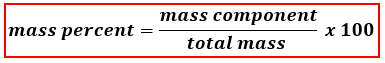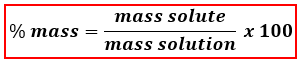# Problem: Calculate the percent by mass of potassium nitrate in a solution made from 45.0g KNO3 and 295 mL of water. Density of water is 0.997 g/mL.A. 15.2%B. 13.3%C. none of themD. 1.51%E. 7.57%

🤓 Based on our data, we think this question is relevant for Professor Olatunde's class at CITYTECH CUNY.

###### FREE Expert Solution

We are asked to calculate for the percent by mass (mass %) of potassium nitrate in solution and to do that we’re going to use the mass percent formula shown below:Since we are dealing with a solution, we can rewrite the equation as:We don’t have the mass of the solution but we can calculate it using the mass of the solute and the mass of solvent.

▪ solute → KNO3
▪ solvent → where KNO3 is dissolved → Water (H2O)

The equation now becomes:###### Problem Details

Calculate the percent by mass of potassium nitrate in a solution made from 45.0g KNO3 and 295 mL of water. Density of water is 0.997 g/mL.

A. 15.2%
B. 13.3%
C. none of them
D. 1.51%
E. 7.57%

What scientific concept do you need to know in order to solve this problem?

Our tutors have indicated that to solve this problem you will need to apply the Mass Percent Formula concept. You can view video lessons to learn Mass Percent Formula. Or if you need more Mass Percent Formula practice, you can also practice Mass Percent Formula practice problems.

What is the difficulty of this problem?

Our tutors rated the difficulty ofCalculate the percent by mass of potassium nitrate in a solu...as medium difficulty.

How long does this problem take to solve?

Our expert Chemistry tutor, Dasha took 2 minutes and 6 seconds to solve this problem. You can follow their steps in the video explanation above.

What professor is this problem relevant for?

Based on our data, we think this problem is relevant for Professor Olatunde's class at CITYTECH CUNY.